# What Are the Different Parts of a Graph?

An error occurred trying to load this video.

Try refreshing the page, or contact customer support.

Coming up next: What is a Linear Equation?

### You're on a roll. Keep up the good work!

Replay
Your next lesson will play in 10 seconds
• 0:05 What is a Grid?
• 0:51 X & Y Axes
• 2:55 Points and Coordinate Pairs
• 5:03 Lesson Summary

Want to watch this again later?

Timeline
Autoplay
Autoplay
Speed

#### Recommended Lessons and Courses for You

Lesson Transcript
Instructor: Elizabeth Foster

Elizabeth has been involved with tutoring since high school and has a B.A. in Classics.

Being able to read a graph isn't just vital for an algebra class. Graphs and charts are used everywhere! We'll take a crash course on the basic x/y plane used in algebra and the fundamental vocab you need.

## What is a Grid?

You've probably used grids before in real life. For example, a chessboard is a grid. Along the bottom, there are columns labeled A through H, and along the side, there are rows labeled 1 through 8. If you want to talk about a specific square on the board, you can refer to it by its number and letter. For example, the pawn here is at B3.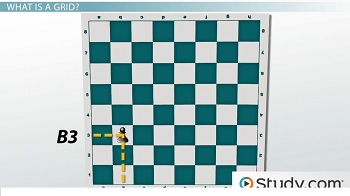The type of grid used in math problems is called the Cartesian plane or xy plane. A Cartesian plane is a little different from a chessboard, but you can think about it the same way. Using a Cartesian plane can help you solve all kinds of math problems and understand the relationships between things in the real world.

In this lesson, you'll learn about the parts of a Cartesian plane, so you'll be all ready to use it when you need to.

## X & Y Axes

On a chessboard, the horizontal location of a piece is labeled with a letter and the vertical location is labeled with a number. On the Cartesian plane, it's a little bit different: both the horizontal and vertical position are labeled with numbers. On a Cartesian plane, you can also have locations that are described with negative numbers.

The numbers that describe a particular location on the Cartesian plane are called coordinates. The x-coordinate of an object on the Cartesian plane tells you how left or right it is from the center of the graph. Every graph has a line called the x-axis, which marks the horizontal location of points on the graph. This is basically a number line, and if you've used number lines before, it should look very familiar.

If you're moving to the left along the x-axis, the number will be negative, and if you're moving to the right, it will be positive. For example, the x-coordinate of this point is 5, because it's 5 units to the right of the center of the graph. The x-coordinate of this point is -5, because it's 5 units to the left of center.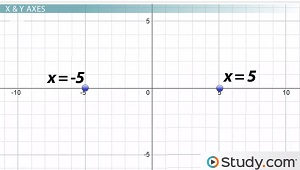Using x-coordinates lets you distinguish between points: for example, Point A in this graph has an x-coordinate of 3, and Point B has an x-coordinate of 5. But how would you distinguish between Point B and Point C? They're obviously not in the same place, but they both have the same x-coordinate!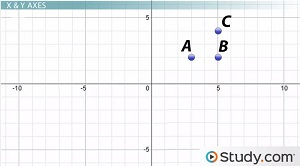That's where the y-coordinates come in. The line that shows the vertical position of points on the graph is called the y-axis. The y-coordinate of an object tells you how far up or down it is on the y-axis, relative to the center of the graph. You can remember which axis is x and which is y by looking at the letters: the letter y has a long tail that goes down, so the y-axis is the axis that goes up and down.

Looking at the y-coordinates lets us describe the differences between these two points. Point B has an x-coordinate of 5 and a y-coordinate of 2. Point C has an x-coordinate of 5 and a y-coordinate of 4.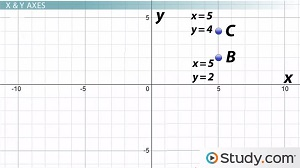## Points & Coordinate Pairs

It's a little bit clunky to walk around saying things like 'This is the point with an x-coordinate of 5 and a y-coordinate of 2,' so we use a shorthand to talk about it. Instead of all that stuff, you can write the numbers as an ordered pair.

An ordered pair is defined as a set of coordinates shown as two numbers inside parentheses. The x-coordinate always comes first, and the y-coordinate always comes second. So the coordinates of Point B on the graph would be (5, 2).

Here are some examples of ordered pairs.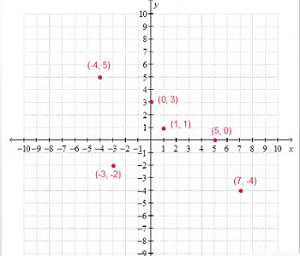A point that's on one of the two axes has a 0 for the other coordinate. For example, this point is labeled (5, 0), because it's 5 units to the right of the middle of the graph, and 0 units up or down. This point is labeled (0, 3), because it's 3 units up from the middle of the graph, and 0 units to the right or left.

The point at the very middle of the graph is called the origin, and its coordinates are (0, 0), because it's 0 units away from the center of the graph in both directions.

To unlock this lesson you must be a Study.com Member.

### Register to view this lesson

Are you a student or a teacher?

### Unlock Your Education

#### See for yourself why 30 million people use Study.com

##### Become a Study.com member and start learning now.
Back
What teachers are saying about Study.com

### Earning College Credit

Did you know… We have over 160 college courses that prepare you to earn credit by exam that is accepted by over 1,500 colleges and universities. You can test out of the first two years of college and save thousands off your degree. Anyone can earn credit-by-exam regardless of age or education level.